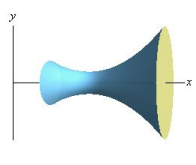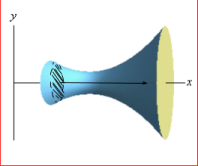×

×

UR / Math / MTH 142 / What is u substitution used for?

# What is u substitution used for? Description

##### Description: -distance and displacement -area -volume -u substitution -work -Hooke's law -Total work
6 Pages 95 Views 3 Unlocks
Reviews

ssudais (Rating: )

Great!

dchen48 (Rating: )

Topics that will be covered:

## What is u substitution used for?-Distance and Displacement

-U substitution

-Area with integrals

-Volume with integrals

-Disk/washer method

-Cylindrical shell method

-Work, Hooke’s Law, Total work against Gravity

*would be a good idea to do previous exams which can be found in the link https://web.math.rochester.edu/courses/current/142/exams/

Distance and Displacement

1. If an object moves with velocity v(x) from x=a to x=b, we have these 2 formulas:

b

a. Displacement = ∫ v(x)dx

a

b

b. Total distance traveled = ∫ |v(x)|dx

## What are the methods to solve for definite integrals?a

2. Keep in kind that when determining total distance traveled, you must find where v(x) is positive and negative, and break it into separate integrals that are added together after integration

U Substitution

1. Essentially using reverse chain rule for integrals that are not simple 2. Substitution rules

1

a. If u=g(x) is differentiable on I and f is continuous on I, then ∫f(g(x)) g (x) dx = ∫f(u)du

b. The process

1 g (x)

1

i. u=g(x), du/dx= g (x) , du= dx

c. You can check by differentiating

## What is the equation for integrals?d. Ex: ∫2x√1 + x dx If you want to learn more check out What does cisgender denote?

2

i. u = 1 + x2

ii. du = 2xdx

iii. Plug in, √udu

iv. ∫u du

1/2

2u2/3+ c If you want to learn more check out What causes patterns in the sky?
Don't forget about the age old question of How party identification affects voting?

v. 3

vi. Answer: (1 ) 23 + x2 3/2 + c

3. Substitution for indefinite integrals

a. Find a function nested in another function

b. u=g(x) (inside function), write everything in terms of u, du

c. Solve the integral in terms of u

d. Go back to x

e. Check by differentiating

ln(x)

f. Ex: ∫ dx

x

i. u = ln(x)

ii. du = 1/x dx

ln(x) If you want to learn more check out What is the function seismology?

iii. ∫ dx x

iv. ∫ud(u)

v. u21 2 + c

vi. Answer: (ln(x)) 21 2 + c

4. Different methods for definite integrals

a. Method 1

i. Solve the indefinite integral first, put it back in terms of x, then go back to definite integral

1

ii. Ex: ∫ e dx , first solve

xx2∫xe dx

x2

0

1. u = x2

2. du=2xdx

3. xdx=½ du Don't forget about the age old question of What do you call the numerical summary of population?

4. ex2= eu

5. ∫xe dx e du = =

x2= 21 u e21 u + c e21 x2+ c If you want to learn more check out What refers to a body of rock and metal?

1

6. Now go back to ∫ e

xx2

0

7. e (plug in 0 and 1) 21 x2∫10

1 − 21 0

8. e e

2

9. 2e − 21

10. 2e−1

iii. With this method

1. Ignore limits of integration until you solve indefinite integral

2. You have to convert back to x before plugging in limits

b. Method 2

i. Change the limits of integration to also be in terms of u

ii. Substitution rule for Definite Integrals

f1 = ∫x=g(b) b

1. ∫ (g(x))g (x)dx (u)du f

x=a

u=g(x)

6

iii. Ex: ∫ dx 1

1

√3x−2

1. u = 3x-2

2. du = 3dx

3. dx = ⅓ du

4. Limits: x=1 → u=1, x=6 →u=16

6

√3x−2= 31∫16

5. ∫ dx du

x=1

u=1

√u

6. 2 (plug in 1 and 16) 32 √u ∫16

u=1

7. ( ) 32 √u − √1

8. (u ) 32 − 1 = 2

iv. With this method

1. Do not need to go back to x at the end, you took care of that when

changing the limits

2. Write the limits at every step

3. If using this method, do not keep writing limits while solving

indefinite integral, this is because if you do write the limits the

intermediate statement will be false and you will forget that you did

not change the limits

Area with Integrals

1. Area of a region bounded by two curves (f(x) and g(x)) on the interval [a,b] can be defined by:

b

a. A = ∫(f(x) (x))dx

− g

a

2. For more complicated ones, use one of the two:

a. A = ∫y(top) − y(bottom)dx

b. A = ∫x(right) − x(left)dy

Volume with Integrals

1. Concept: problems give a few functions and ask for you to find the volume when it is rotated around an axis or another function

a. This is an example of what a problem would look like in concept

b.2. The idea is that you can slice such a figure into a bunch of 2-dimensional cross sections and drag it across the interval to get the volume, like so:

a.3. Thus this is a basic equation for volume:

a. V = ∫cross − sectional area

4. The main methods used are disk/washer or cylindrical shell

Disk/Washer Method

1. This method simply takes the cross-sectional area of the figure perpendicular to the axis of revolution

a.b. This is an example, as it is rotated about the x-axis and the cross-sectional area is perpendicular to the x-axis

2. The numerical equation is:

a. V = π∫r outer inner dx or dy

2 − r2

b. If the axis is horizontal, integrate with respect to dx

c. If the axis is vertical, integrate with respect to dy

Cylindrical shell method

1. This method takes the cross-sectional area of the figure parallel to the axis of revolution 2. The numerical equation is:

a. V = 2π(radius)(height) dx or dy

b. If the axis is horizontal, integrate with respect to dy

c. If the axis is vertical, integrate with respect to dx

Work, Hooke’s Law, Total work against Gravity

1. Work is denoted by the equation W=Fd, where F=constant force and d=distance a. However you can use integrals to find the work needed to move an object over an interval where f(x)=force:

i. W = ∫f(x)dx

b. The units of measurement are Joules (J) and Newton meters (1 to 1 conversion) i. A newton is a kg times acceleration due to gravity ( m/s )2

2. Hooke’s Law

a. This law states that the force needed to extend or compress a spring by some distance x, scales linearly with respect to that distance. Numerically, this is denoted as f(x)=kx

b. Most integrals with this law are set up as so:

i. W = ∫(kx)dx

c. Most questions revolve around finding the k in order to calculate the work needed over a certain interval

3. Total work against Gravity

a. These are problems such as ones involving cables or pumping fluid b. This is a bit more complex than work problems because the movement is not restricted to a single dimension, rather we must cut up what we want to measure into cross-sectional areas

c. The general equation:

i. Total Work = ∫(distance traveled by slice)(weight of slice)

d. How to get “weight of slice”

i. Cable problems

1. Multiply density of cable (in whatever unit) by dx (or whatever unit) ii. Pumping problems

1. Multiply density of liquid (in whatever unit) by the cross-sectional

area also by dx

2. If given unit is only mass, then must multiply by g, the gravitational constant

Page ExpiredIt looks like your free minutes have expired! Lucky for you we have all the content you need, just sign up here